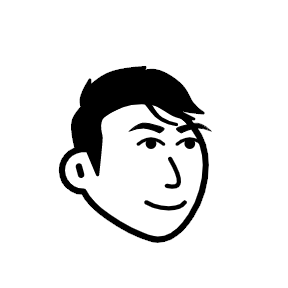7:40 AM
A new tag has been created, it already has 5 questions:
0In a triangle $ABC$, the bisectors of angles $B$ and $C$ lie on lines $x=y$ and $y=0$.If $A$ is $(1,2)$ then equation of $BC$ is?

-1I already saw this question here, but the answers are not clear for me. Hope I could get clear solution and answer. I already got the $2^{nd}$ vertex which is $(4, 5)$. How do I get the other two vertices? Thank you!

1I want to know what is the condition for two lines to be coplanar . I searched it on internet. I found that for coplanar the scalar product should be zero . But I could not understand why it should be zero . And what are the three vectors whose scalar product is zero

-4If the lines $x-2=y-3=\frac{4-z}{k}$ and $\frac{x-1}{k}=\frac{y-4}{2}=z-5$ are coplanar , then $k$ have how many values? And write the values.

1Let point $P= (5,3)$ and a point $R$ on $y=x$ and $Q$ on the $x$ axis be such that $PQ+QR+RP$ is minimum. Then the coordinates of $Q$ are?

The tags and were rejected before. I do not see much difference between these tags and tag.
7Quite recently (it can still be seen in the list of new tags) the tag line was created. (As far as I can say, it was created in this post. Or at least it was the first post using this tag that I saw. It was later removed from that post, but added to other posts before it was removed from the syst...

6 hours later…2:11 PM
The tag has been created here:
0Let $f$ be entire such that $\forall |z|\geq1$, $|f(z)|\leq e^{-|z|}$. Then how do we prove that $f$ is constant? Can we map the complement of unit disk conformally onto the complex plane or the unit disk, by virtue of which we may then invoke Liouville's Theorem?Any ideas. Thanks beforehandLiouville's theorem (complex analysis)
In complex analysis, Liouville's theorem, named after Joseph Liouville, states that every bounded entire function must be constant. That is, every holomorphic function f for which there exists a positive number M such that | f ( z ) | ≤ M {\displaystyle |f(z)|\leq M} for all z {\displaystyle z} in C {\displaystyle \mathbb {C} } is constant. Equivalently...

2 hours later…4:30 PM
I suppose that 2017 thread will be created soon, but here are the most recent entries in Tag management 2016:
3Could we pluralize quiver? (Previously requested on: Tag management 2015)

2How about we pluralize comment? Currently the plural form is a synonym of the singular form. But if we agree on pluralized tags, then this should certainly be reversed.

1I'm not seeing the point of gmat-exam; is there any good reason to keep this around?

0I think (fractal-analysis) and (fractals) could be merged. The first one is barely used & almost all of it's question has the second one as a duplicated tag.5:03 PM
BTW now there is tag-excerpt and tag-wiki for tag.

1 hour later…6:04 PM
4In topology, several separation axioms (such as Hausdorff, completely regular, normal, ...) and countability axioms (such as first countability or separability) are often used ant there are also some questions about them on this site. Hence it is not entirely surprising that we also have tags sep...

The outcome of the above discussion seems to be removal of tag (and replacing it by more specific tags). Brian M. Scott's answer got 5 upvotes. As the question is more than two weeks old, it is no longer shown in the , so it does not seem likely that there will be any additional feedback.
I have already created tag (with a very basic tag-excerpt and tag-wiki) and also tag (here is tag-excerpt and tag-info.)
I plan to start retagging at a reasonable place. I suppose that in the most of the questions one of the newly created tags or tag will be used.
Currently there are 30 questions tagged - I will list them here, ordered by id: